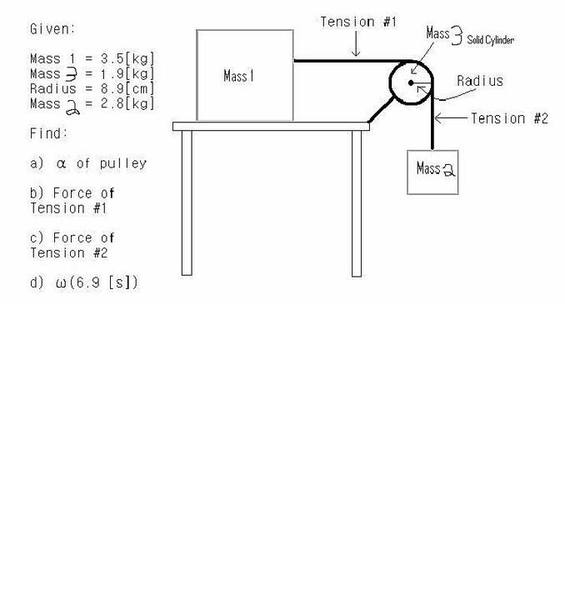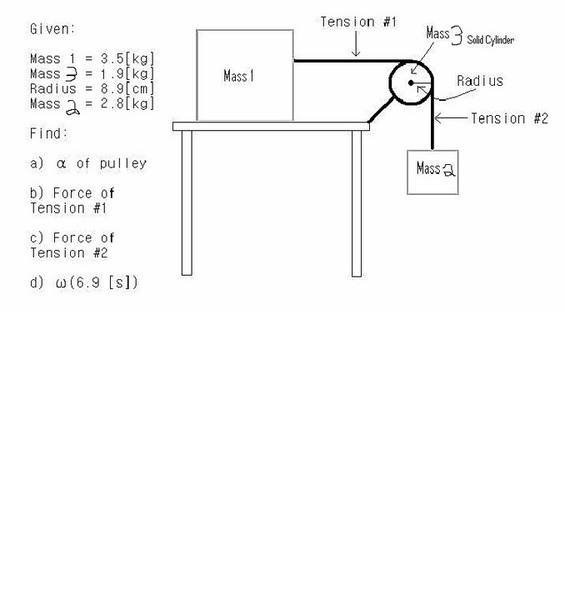# Two boxes connected with a pulley, one hanging

Two boxes connected with a pulley, one hanging *updated*

## Homework Statement

There is a Mass (m1) on a table. The coefficient of friction between the table and m1 is 0.18. The second mass (m2) is hanging, suspended by a string around a pulley (m3) attached to m1. The pulley has a radius (R) and is considered a solid cylinder.
m1=3.5[kg]
m2=2.8[kg]
m3=1.9[kg]
R=0.089[m]

Find:
a) alpha of the pulley
b.)Ft1
c.)Ft2
d.)omega @ (6.9)

## Homework Equations

I for solid cylinder = (1/2)M2R2
Fnet=ma
Torquenet=I*alpha
alpha=a/R

## The Attempt at a Solution

I drew a torque fbd for the pulley, I found I for the pulley, I drew fbd's for the boxes, got my equations like

m1-->
X) Ft1-Ff=m1a
Y) Fn-Fg=0

Fn=m1g
Ft1=m1a+Ff
Ft1=m1a+Mew*m1*g

m2--->
Y) Ft2-Fg=m2(-a)
Ft2=-m2a+m2g
and finally

Torquenet=I*alpha so
Torque1=R*sin(90)*Ft1 = R*Ft1 (k)
Torque2=R*sin(90)*Ft2 = R*Ft2 (-k)
==>R*Ft1-R*Ft2=I*alpha

and alpha= a/R

So
R*(m1a+Mew*m1*g)-R*(-m2a+m2g)=((1/2)M3R2)*(a/R)

That equation just has one uknown --> a

For a i got 3.97[m/s2]
Can someone spot what, if anything, I'm doing something wrong?

EDIT: Sorry for the mistakes..

Last edited:

Doc Al
Mentor
It would be easier to spot an error if you showed your three primary equations (for m1, m2, and m3) and your solution for acceleration symbolically (without numerical values plugged in).

Doc Al
Mentor
Which is which? Is m3 the pulley or the hanging mass?

nrqed
Homework Helper
Gold Member
Which is which? Is m3 the pulley or the hanging mass?

The question identifies the masses. m3 is the pulley

nrqed
Homework Helper
Gold Member

## Homework Statement

There is a Mass (m1) on a table. The coefficient of friction between the table and m1 is 0.18. The second mass (m2) is hanging, suspended by a string around a pulley (m3) attached to m1. The pulley has a radius (R) and is considered a solid cylinder.
m1=3.5[kg]
m2=2.8[kg]
m3=1.9[kg]
R=0.089[m]

Find:
a) alpha of the pulley
b.)Ft1
c.)Ft2
d.)omega @ (6.9)

## Homework Equations

I for solid cylinder = (1/2)M2R2
Fnet=ma
Torquenet=I*alpha
alpha=a/R

## The Attempt at a Solution

I drew a torque fbd for the pulley, I found I for the pulley, I drew fbd's for the boxes, got my equations like

m1-->
X) Ft1-Ff=m1a
Y) Fn-Fg=0

Fn=m3g

It should be m_1 there

The question identifies the masses. m3 is the pulley

unfortunatly in my following equations though, I treated m3 as the mass used in Force of tension 2, where that should have been m2..

I'm starting to screw myself up now, after solving for a from that last equation I wrote up there, I got a different value for a then I originally got..

Then I realized that Ft1 is negative on my fbd, so I tried changing that and I got a totally different number that no one else has.

All I have to do is find my three equations, and solve for a right?? Why is this proving to be so difficult for me..

nrqed
Homework Helper
Gold Member

Torquenet=I*alpha so
Torque1=R*sin(90)*Ft1 = R*Ft1 (k)
Torque2=R*sin(90)*Ft2 = R*Ft2 (-k)
==>R*Ft1-R*Ft2=I*alpha
To make my point more clear: the rotation is clockwise, so the angular rotation is
alpha (-k), not alpha k . This is why we end up with

R*Ft1-R*Ft2=- I*alpha

or

-R*Ft1+R*Ft2= I*alpha

Last edited:

You are on the right track!! Don't get discouraged, you are almost done.

Looks to me like it should be -Ft1+R*Ft2= I alpha
since the mass m2 is falling, the torque on that side is larger than the torque from T1

Ok so with my original equation where I had Ft1 as a positive direction, the equation I got for a was this:

a=(g(m2-Mew*m1))/(m1+m2-(m3/2))

After changing the direction of Ft1 I get a is equal to

a= (-g(Mew*m1-m2))/((m3/2)-m1-m2)

They both result with a value of 3.97[m/s2] but the second equation results in a negative value.. doesn't that mean that one of my directions is off if the value is negative?

nrqed
Homework Helper
Gold Member

Ok so with my original equation where I had Ft1 as a positive direction, the equation I got for a was this:

a=(g(m2-Mew*m1))/(m1+m2-(m3/2))

After changing the direction of Ft1 I get a is equal to

a= (-g(Mew*m1-m2))/((m3/2)-m1-m2)

They both result with a value of 3.97[m/s2] but the second equation results in a negative value.. doesn't that mean that one of my directions is off if the value is negative?

There is something fishy going on, indeed. Give me a minute to double check everything.
Meanwhile, can you check carefully the question and make sure that it is exactly what you wrote in your post? Thanks

nrqed
Homework Helper
Gold Member

Ok so with my original equation where I had Ft1 as a positive direction, the equation I got for a was this:

a=(g(m2-Mew*m1))/(m1+m2-(m3/2))

After changing the direction of Ft1 I get a is equal to
Wait...you have to change the sign of both Ft1 and Ft2 in your torque equation, not just one

nrqed
Homework Helper
Gold Member

Using

$$R T_2 - R T_1 = \frac{1}{2} m_3 R^2 \frac{a}{R}$$

and using the same T2 and T1 you had, I find

$$a = \frac{m_3 g - \mu m_1 g}{m_3/2+m_1 + m_2 }$$

Last edited:

There is something fishy going on, indeed. Give me a minute to double check everything.
Meanwhile, can you check carefully the question and make sure that it is exactly what you wrote in your post? ThanksLast edited:

Wait...you have to change the sign of both Ft1 and Ft2 in your torque equation, not just one

Why do I have to change the sign for Ft2? Its positive in the fbd for m3 and the torque from Ft2 is still out of the board (-)

nrqed
Homework Helper
Gold Memberplease note that I changed the pulley to m3 and the hanging mass to m2 when I posted; I forget why.

AHHH!!!!!
And where do they give the coefficient of friction?

In any case, the formula is

$$a = \frac{ m(hanging) g - \mu m(on \,table) g}{m(pulley)/2 + m(on \,table) + m(hanging)}$$

nrqed
Homework Helper
Gold Member

Why do I have to change the sign for Ft2? Its positive in the fbd for m3 and the torque from Ft2 is still out of the board (-)

I mean that the correct equation is

Ft2 - Ft1 = I alpha

I mean that the correct equation is

Ft2 - Ft1 = I alpha

I dont get how Ft2 is positive now though. Isn't it (-k) direction (so negative in torque equation) and positive in the fbd for m3?

nrqed
Homework Helper
Gold Member

I dont get how Ft2 is positive now though. Isn't it (-k) direction (so negative in torque equation) and positive in the fbd for m3?

This is what I explained in post number 7

The angular acceleration is clockwise. This means that the angular acceleration is alpha(-k)

So we get

T2 (-k) + T1 (k) = I alpha (-k)

therefore

-T2 + T1 = - I alpha

or

T2 - T1 = I alpha

This is what I explained in post number 7

The angular acceleration is clockwise. This means that the angular acceleration is alpha(-k)

So we get

T2 (-k) + T1 (k) = I alpha (-k)

therefore

-T2 + T1 = - I alpha

or

T2 - T1 = I alpha
Oh..

Sorry, I didn't see post 7 somehow. Ok so that makes sense. I thought alpha could be positive and negative, infact I believed it must be able to be +or- but a peer said no. lol.. so it kind of escaped me..

Thank you so much.. So Ft1 will be = -m1a-Mew*m1g

because Ft1 itself is negative in the fbd for m3..

so with the negative Ft1 in the torque equation, that will distribute out and make those two factors positive (in Ft1 itself)

nrqed
Homework Helper
Gold Member

Oh..

Sorry, I didn't see post 7 somehow. Ok so that makes sense. I thought alpha could be positive and negative, infact I believed it must be able to be +or- but a peer said no. lol.. so it kind of escaped me..
well, one has to be very careful about the language used. To be exact, alpha itself is always positive (it's the magnitude of the angular acceleration). But the components of the angular acceleration vector may be negative. Note that when I write alpha (-k), the value of alpha itself is positive.
Thank you so much.. So Ft1 will be = -m1a-Mew*m1g

because Ft1 itself is negative in the fbd for m3..

so with the negative Ft1 in the torque equation, that will distribute out and make those two factors positive (in Ft1 itself)

not quite. T1 is positive. And it is given by T1 = m1 a + mu m1 g, as you had initially written.

well, one has to be very careful about the language used. To be exact, alpha itself is always positive (it's the magnitude of the angular acceleration). But the components of the angular acceleration vector may be negative. Note that when I write alpha (-k), the value of alpha itself is positive.

not quite. T1 is positive. And it is given by T1 = m1 a + mu m1 g, as you had initially written.

Ok I got myself all mixed up... I'm on track now lol

Again, thanks so much..

oh, and the picture for some reason doesn't include the Mew, I apologize, but the written part of the question does.

So I get a=2.93 [m/s2]